﻿ Excel vba 实例（8）- 利用正则表达式进行定向提取 - 永恒君的百宝箱

# Excel vba 实例（8）- 利用正则表达式进行定向提取

2675℃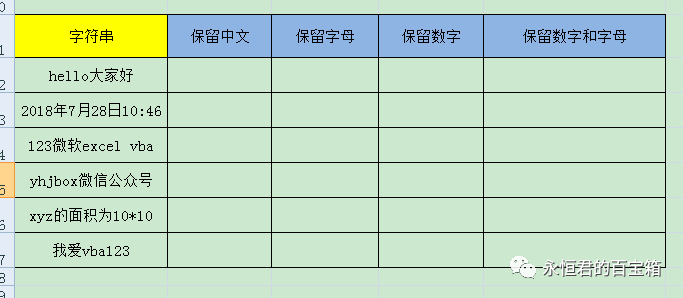1、需要新建模块，把代码写在其中。不能直接写在sheet表中。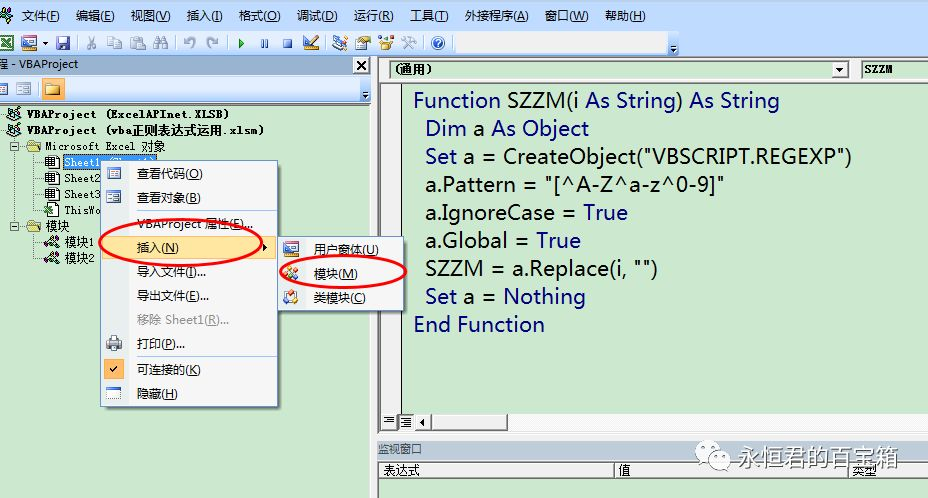2、代码开头的ZW、SZ、ZM、SZZM即为自定义的函数名，都是各自独立的函数，调用的时候就是写它们。

'只保留中文的代码如下:

Function ZW(i As String) As String

Dim a As Object

Set a = CreateObject("VBSCRIPT.REGEXP")

a.Pattern = "[^\u4e00-\u9fa5]"

a.IgnoreCase = True

a.Global = True

ZW = a.Replace(i, "")

Set a = Nothing

End Function

'只保留数字的代码如下:

Function SZ(i As String) As String

Dim a As Object

Set a = CreateObject("VBSCRIPT.REGEXP")

a.Pattern = "[^0-9]"

a.IgnoreCase = True

a.Global = True

SZ = a.Replace(i, "")

Set a = Nothing

End Function

'只保留字母的代码如下:

Function ZM(i As String) As String

Dim a As Object

Set a = CreateObject("VBSCRIPT.REGEXP")

a.Pattern = "[^A-Z^a-z]"

a.IgnoreCase = True

a.Global = True

ZM = a.Replace(i, "")

Set a = Nothing

End Function

'只保留数字和字母的代码如下:

Function SZZM(i As String) As String

Dim a As Object

Set a = CreateObject("VBSCRIPT.REGEXP")

a.Pattern = "[^A-Z^a-z^0-9]"

a.IgnoreCase = True

a.Global = True

SZZM = a.Replace(i, "")

Set a = Nothing

End Function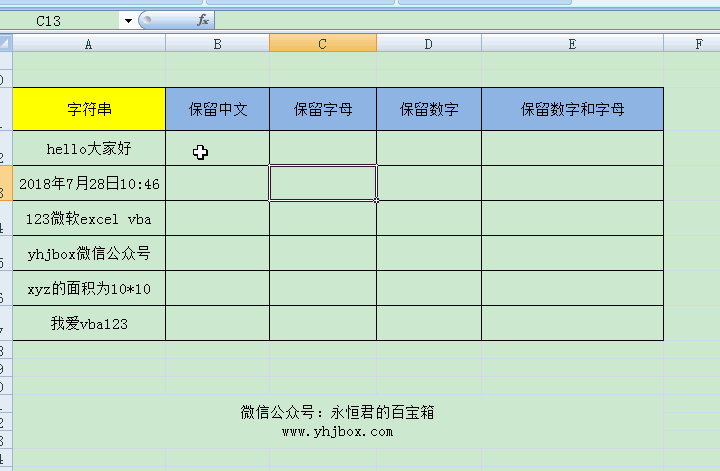Excel VBA功能很强大，但是要熟练的运用起来还是有一定的难度，好在永恒君帮大家搜集了几套关于VBA的视频教程，非常的实用。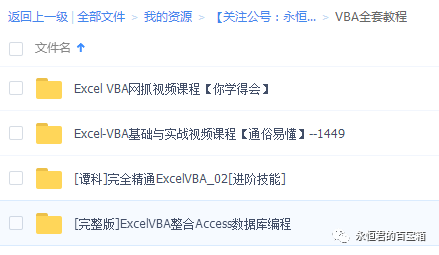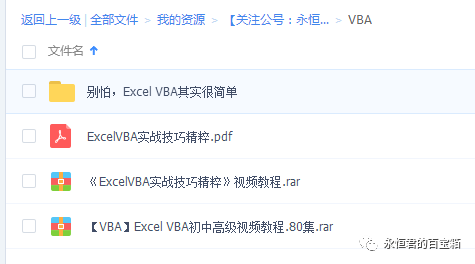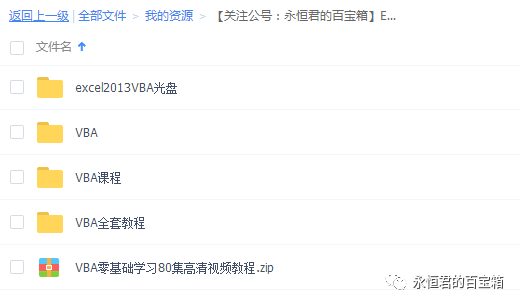(2)个小伙伴在吐槽
1. VBA
匿名2019-04-16 13:40 回复
• 在微信公众号“永恒君的百宝箱”里回复
永恒君2019-04-23 10:32 回复